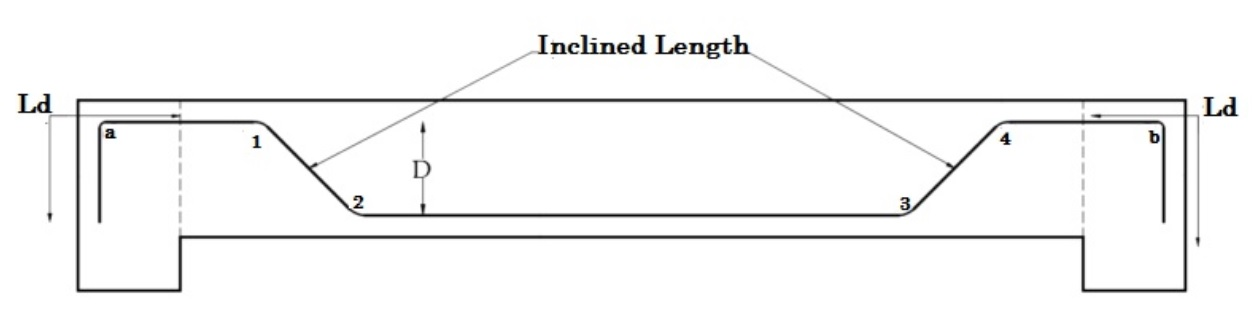# How to Calculate Cutting Length of Bent Up Bar in Slab | Bar Bending Schedule | Crank Bar:

How to Cutting Length of Bent Up Bar in Slab | Cutting Length of Crank Bar | Bar Bending Schedule | Cutting Length Formula, commonly referred to as “BBS” is a comprehensive list that describes the location, mark, type, size, length and number, and bending details of each bar or fabric in a Reinforcement Drawing of a Structure.

This process of listing the location, type and size, number of and all other details is called “Scheduling”. In context of Reinforcement bars, it is called bar scheduling. In short, Bar Bending Schedule is a way of organizing rebars for each structural unit, giving detailed reinforcement requirements.

## What is Cutting Length?

What is cutting length | length of Steel which is provided in slab and cut into desire length according to requirement of design of slab is known as cutting length. Cutting Length formula

## How to Calculate Cutting Length of Bent Up Bar in Slab or Crank Bar in Slab?

As a site engineer, you need to calculate the cutting length of bars according to the slab dimensions and give instructions to the bar benders.

[wpdm_package id=’2594′]

For small area of construction, you can hand over the reinforcement detailing to the bar benders. They will take care of cutting length. But beware, that must not be accurate. Because they do not give importance to the bends and cranks. They may give some extra inches to the bars for the bends which are totally wrong. So it is always recommended that as a site engineer calculate the cutting length yourself. In this article, we will discuss how to the calculate length for reinforcement bars of slab. Let’s start with an example.

Where, Cutting Length formula

Diameter of the bar = 12 mm

Clear Cover = 25 mm

Clear Span (L) = 8000

Slab Thickness = 200 mm

Development Length(Ld) = 40d

Cutting Length = Clear Span of Slab + (2 x Development Length) + (2 x inclined length) – (45° bend x 4) – (90° bend x 2)Cutting Length of Bent Up Bar in Slab | Bar Bending Schedule | Cutting Length of Crank Bar | What is Cutting Length | Cutting Length formula

Inclined length = D/(sin 45°) – dD/ (tan 45°) = (D/0.7071) – (D/1)= (1D – 0.7071D)/0.7071= 0.42 D

As you can see there are four 45°bends at the inner side (1,2,3 & 4) and two 90° bends ( a,b ).

45° = 1d ; 90° = 2d

Cutting Length = Clear Span of Slab + (2 X Ld) +(2 x 0.42D) – (1d x 4) – (2d x 2) [BBS Shape Codes]

Where,

d = Diameter of the bar.

Ld = Development length of bar.

D = Height of the bend bar.

In the above formula, all values are known except ‘D’.

So we need to find out the value of “D”.

D = Slab Thickness – (2 x clear cover) – (diameter of bar)

= 200 – (2 × 25) – 12

= 138 mm

Now, putting all values in the formula

Cutting Length = Clear Span of Slab + (2 x Ld) +(2 x 0.42D) – (1d x 4) – (2d x 2)

= 8000 + (2 x 40 x 12) +(2 x 0.42 x 138) – (1 x 12 x 4) – (2 x 12 x 2)

Cutting Length = 8980 mm or 8.98 m.

So for the above dimension, you need to cut the main bars 8.98 m in length.

### Conclusion:

Full article on How to Calculate Cutting Length of Bent Up Bar in Slab | Bar Bending Schedule. Thank you for the full reading of this article in “The Civil Engineering” platform in English. If you find this post helpful, then help others by sharing it on social media. For More detail please see the above video tutorial further you have any question regarding article please tell me in comments.

1.महेंद्र सिंह